# Determine 2-'{F} 78 +93s + 256 F(s) (5-4)(s? +65 +34) Click here to view the table...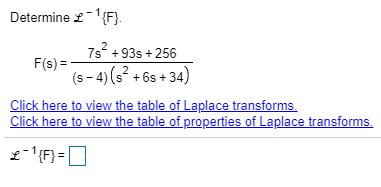Determine 2-'{F} 78 +93s + 256 F(s) (5-4)(s? +65 +34) Click here to view the table of Laplace transforms. Click here to view the table of properties of Laplace transforms. 2-{F}=0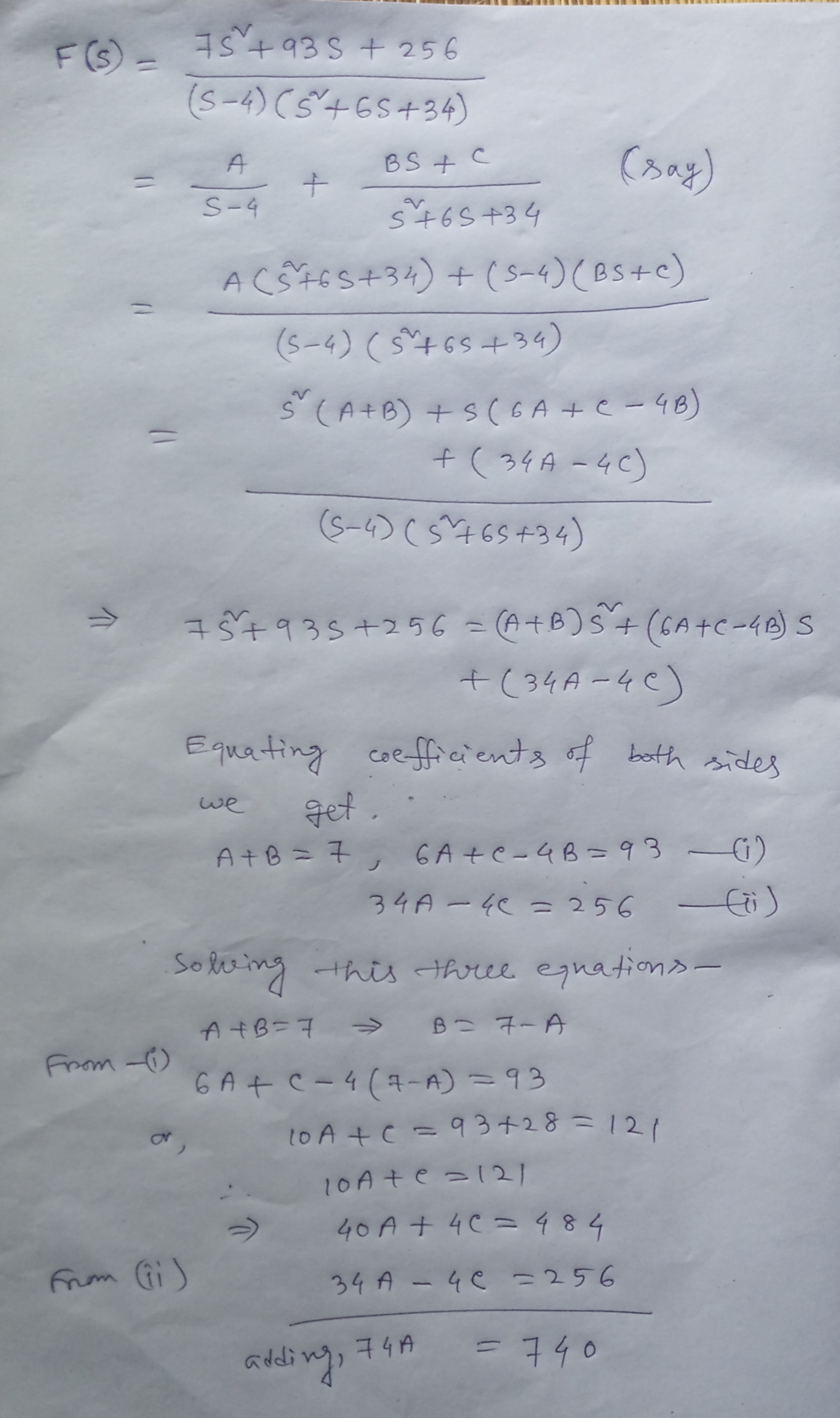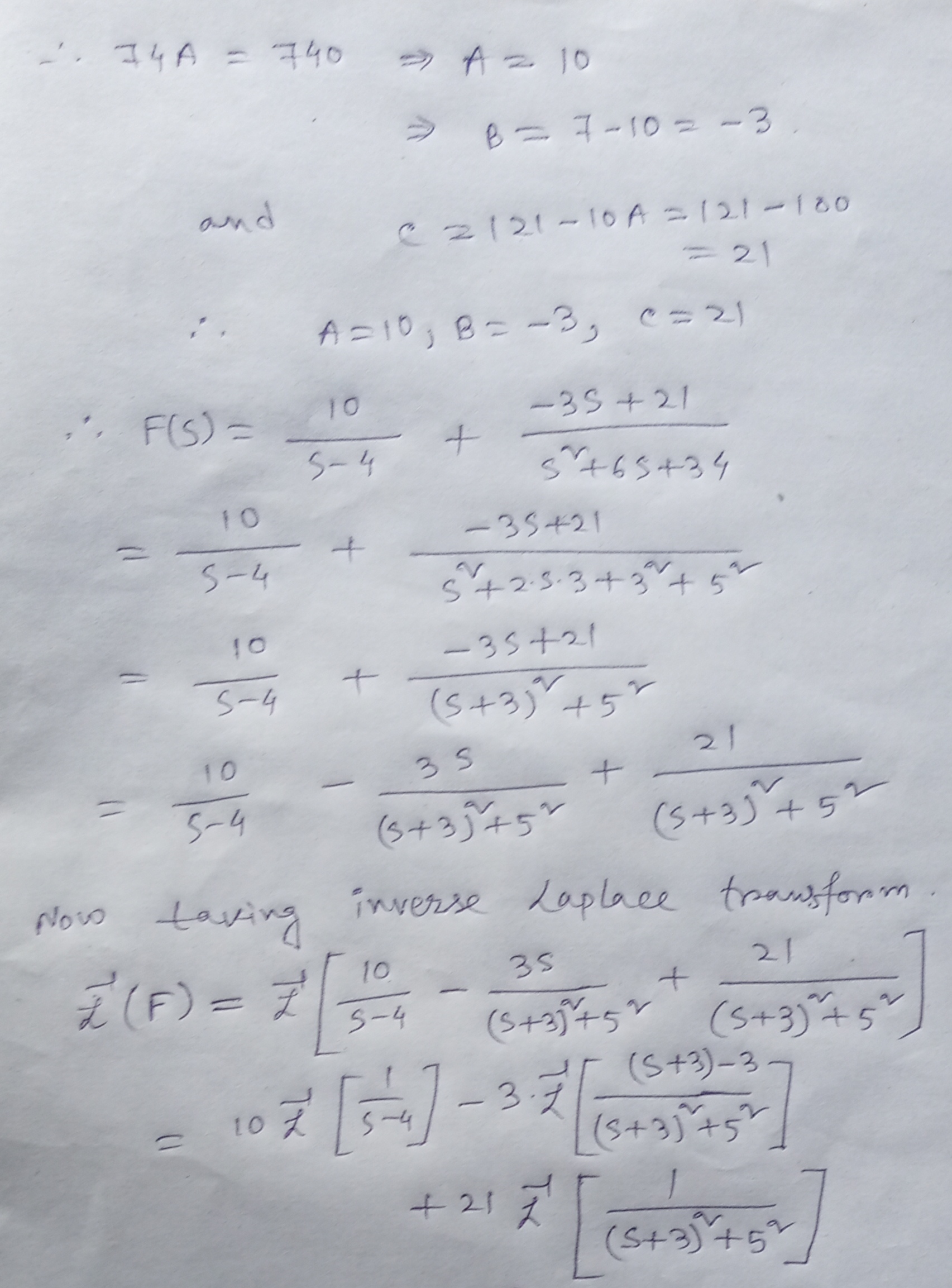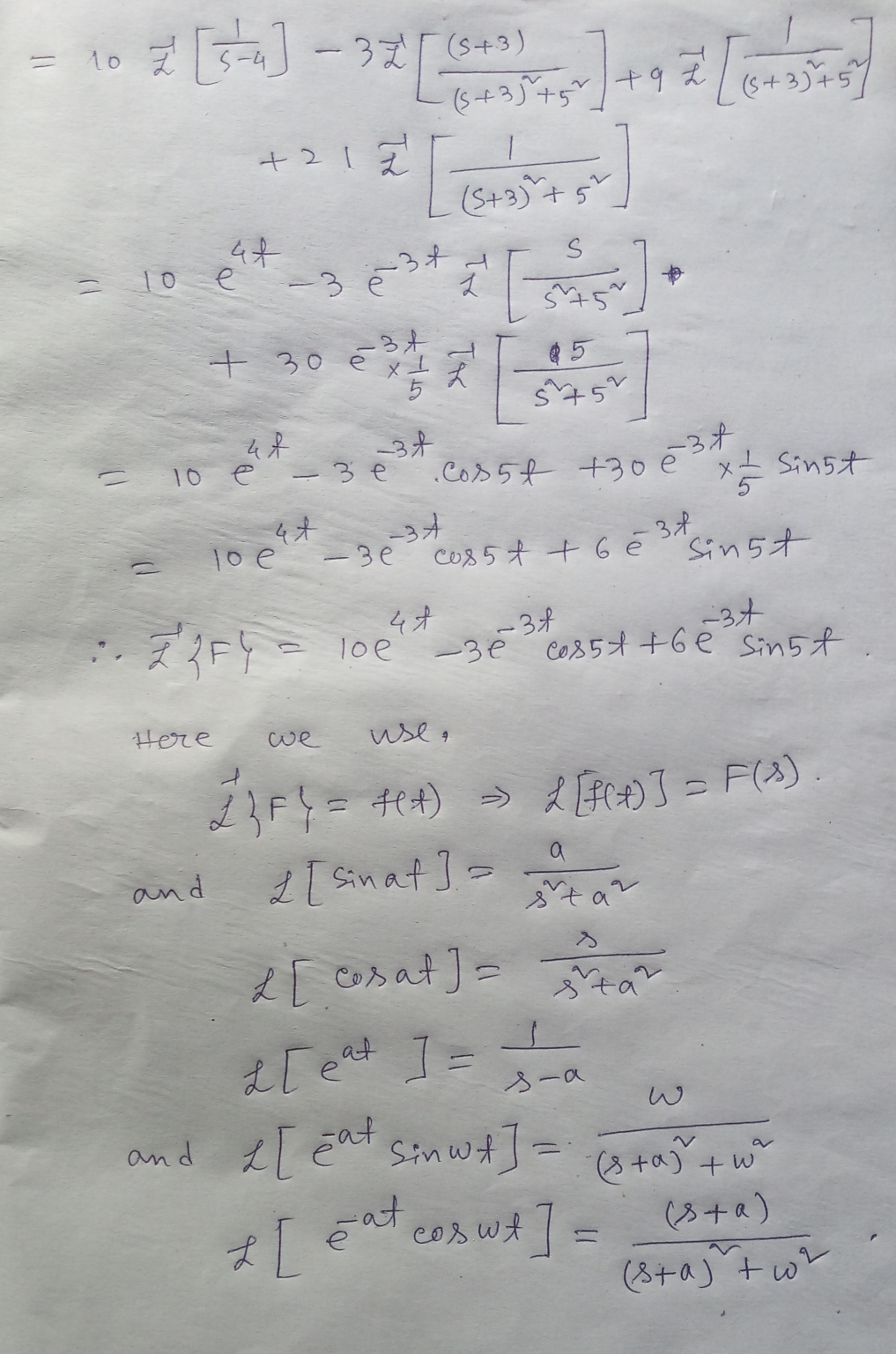Similar Homework Help Questions
• ### Determine £-1 {F) 2s2+5 s2 F(s) + sF(s)-12F(S)-2 Click here to view the table of Laplace...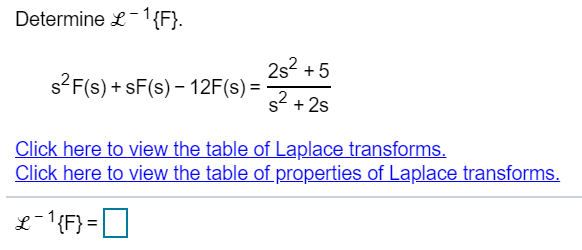Determine £-1 {F) 2s2+5 s2 F(s) + sF(s)-12F(S)-2 Click here to view the table of Laplace transforms Click here to view the table of properties of Laplace transforms Determine £-1 {F} 4s +4 s2 +10s +25 Click here to view the table of Laplace transforms Click here to view the table of properties of Laplace transforms

• ### Determine 2-1{F} -45² - 185-2 F(s) = (s+5)(s + 2) Click here to view the table...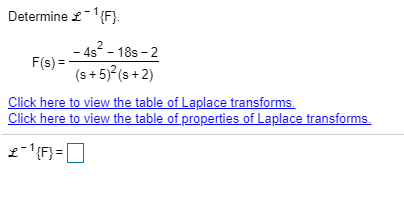Determine 2-1{F} -45² - 185-2 F(s) = (s+5)(s + 2) Click here to view the table of Laplace transforms. Click here to view the table of properties of Laplace transforms. 2-{F}=0

• ### Determine L '{F}. F(s) = 882 - 145 +4 s(s - 5)(-4) Click here to view...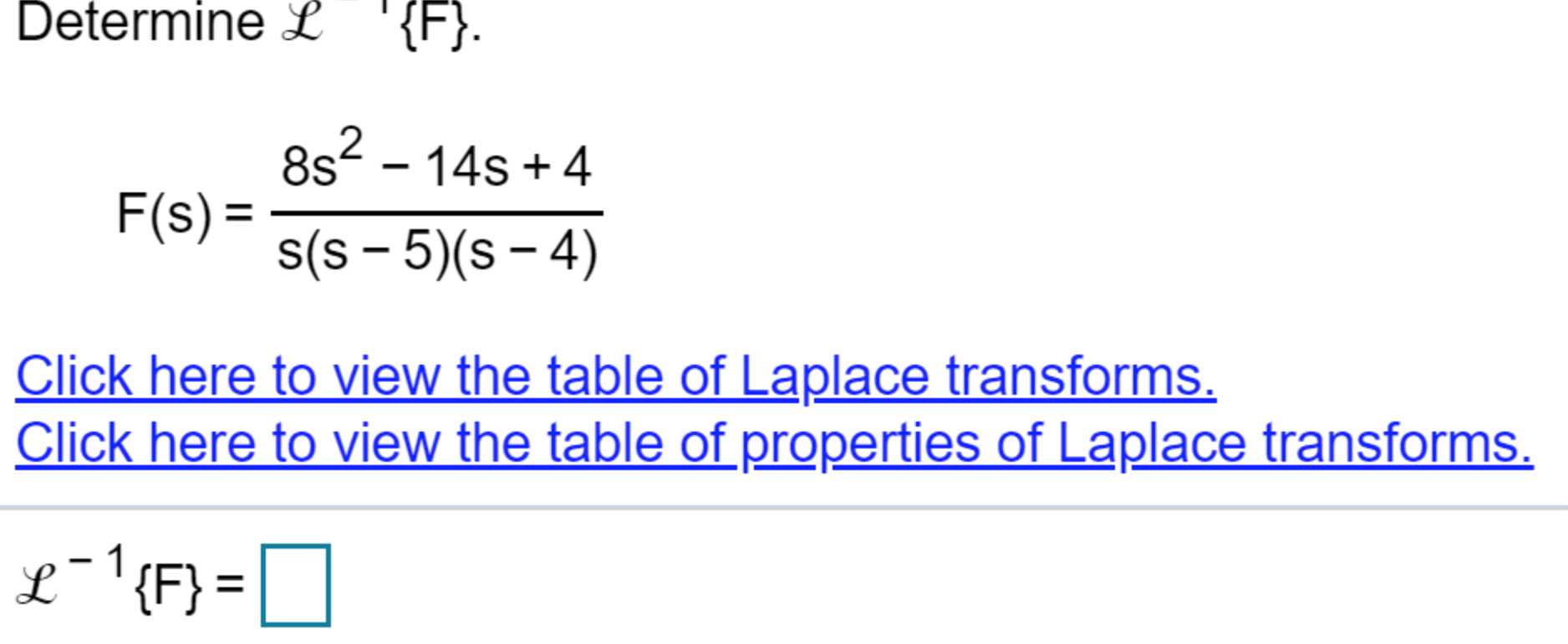Determine L '{F}. F(s) = 882 - 145 +4 s(s - 5)(-4) Click here to view the table of Laplace transforms. Click here to view the table of properties of Laplace transforms. 1 L-'{F}=0

• ### Determine {F} = 45 + 315 +66 F(s) = (5+4)(s +6) Click here to view the...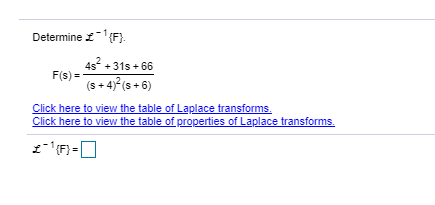Determine {F} = 45 + 315 +66 F(s) = (5+4)(s +6) Click here to view the table of Laplace transforms. Click here to view the table of properties of Laplace transforms.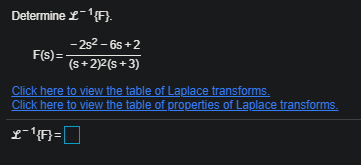Determine L-'{F} F(s)= -252-6s+2 (s+2)(8+3) Click here to view the table of Laplace transforms. Click here to view the table of properties of Laplace transforms. L-'{f}=0

• ### Determine - (F) - 352-258-26 F(s) (s + 2)2(+6) Click here to view the table of...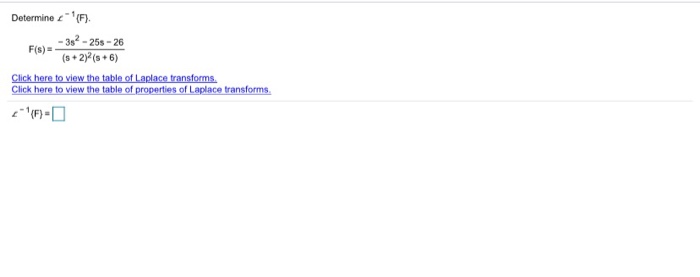Determine - (F) - 352-258-26 F(s) (s + 2)2(+6) Click here to view the table of Laplace transforms. Click here to view the table of properties of Laplace transforms.

• ### Determine 2 - {F} -352 - 198 - 12 F(s) = (s +6)2(5+3) Click here to...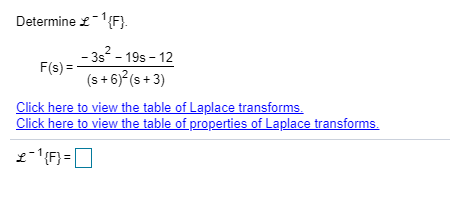Determine 2 - {F} -352 - 198 - 12 F(s) = (s +6)2(5+3) Click here to view the table of Laplace transforms. Click here to view the table of properties of Laplace transforms. 2-'{F}=0

• ### Determine &-'{F}. 332 +33s + 74 F(s)= (s +4)2 (s + 2) + Click here to...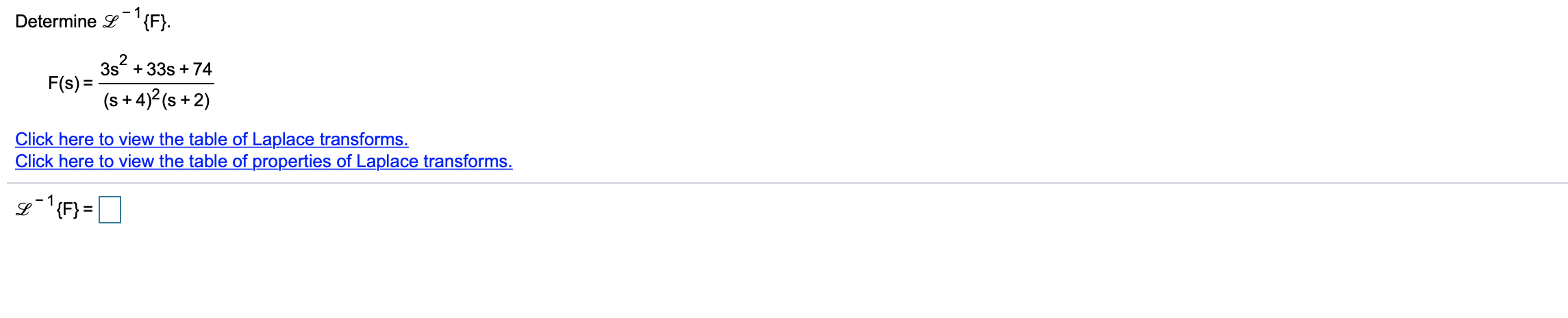Determine &-'{F}. 332 +33s + 74 F(s)= (s +4)2 (s + 2) + Click here to view the table of Laplace transforms. Click here to view the table of properties of Laplace transforms. \$1 {F} =

• ### Determine 2-1{F}. -482-22s +7 F(s) = (s +6)2 (s + 1) Click here to view the...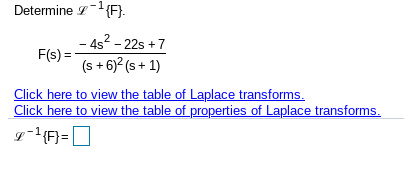Determine 2-1{F}. -482-22s +7 F(s) = (s +6)2 (s + 1) Click here to view the table of Laplace transforms. Click here to view the table of properties of Laplace transforms. e-1{F}=0

• ### Determine L-'{F} F(s) = 2 5sº - 13s +6 s(s - 3)(s - 2) Click here...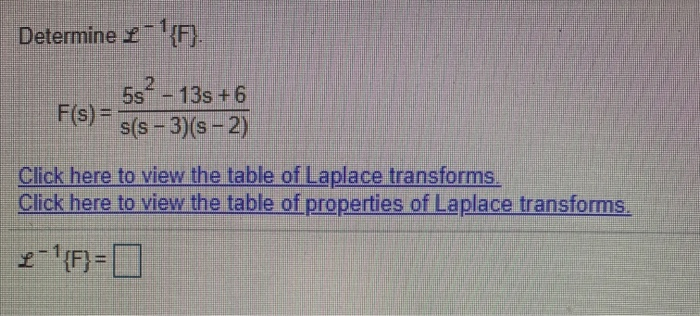Determine L-'{F} F(s) = 2 5sº - 13s +6 s(s - 3)(s - 2) Click here to view the table of Laplace transforms. Click here to view the table of properties of Laplace transforms. 2-'{F}=0

Free Homework App